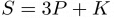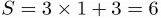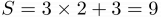## Problem J4: Big Bang Secrets

Sheldon and Leonard are physicists who are fixated on the the BIG BANG theory. In order to exchange secret insights they have devised a code that encodes UPPERCASE letters by shifting their letters forward.

Shifting a letter by S positions means to go forward S letters in the alphabet. For example, shifting B by S = 3 positions gives E. However, sometimes this makes us go past Z, the last letter of the alphabet. Whenever this happens we wrap around, treating A as the letter that follows Z. For example, shifting Z by S = 2 positions gives B.

Sheldon and Leonard's code depends on a parameter K and also varies depending on the position of each letter in the word. For the letter at position P, they use the shift value of$S = 3P + K$.

For example, here is how ZOOM is encoded when K = 3. The first letter Z has a shift value of$S = 3 \times 1 + 3 = 6$; it wraps around and becomes the letter F. The second letter, O, has$S = 3 \times 2 + 3 = 9$ and becomes X. The last two letters become A and B. So Sheldon sends Leonard the secret message: FXAB

Write a program for Leonard that will decode messages sent by Sheldon.

### Input Format

The input will be two lines. The first line will contain the positive integer K (K < 10), which is used to compute the shift value. The second line of input will be the word, which will be a sequence of consecutive uppercase characters of length at most 20.

### Output Format

The output will be the decoded word of uppercase letters.

### Sample Input 1

3
FXAB

### Sample Output 1

ZOOM

### Sample Input 2

5
JTUSUKG

### Sample Output 2

BIGBANG

Point Value: 5
Time Limit: 2.00s
Memory Limit: 16M